# Civil Engineering - UPSC Civil Service Exam Questions

31.

Consider the following statements in relation to the given sketch :
1. Soil is partially saturated at degree of saturation = 60%
2. Void ratio = 40%
3. Water content = 30%
4. Saturated unit weight = 1.5 g/cc
Of these statements :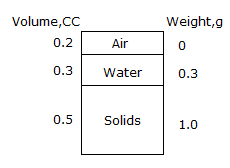A. 1, 2 and 3 are correct B. 1, 3 and 4 are correct C. 2, 3 and 4 are correct D. 1, 2 and 4 are correct

Explanation:

No answer description available for this question. Let us discuss.

32.

A journey from work to home made by walking to the bus, travelling by bus to the station and completing the journey by train is regarded as

 A. 4 trips B. 3 trips C. 2 trips D. 1 trip

Explanation:

No answer description available for this question. Let us discuss.

33.

If P is the direct load, L is the length of the member, A is the uniform area of cross-section and E is the Young's modulus; then strain energy due to direct stress caused by a gradually applied load is

 A.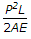B.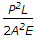C.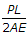D.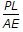Explanation:

No answer description available for this question. Let us discuss.

34.

A T-section is used as a simply supported beam with uniform loading. The maximum bending stress for a given load will occur at the

 A. top of the section B. C.G. of the section C. mid-point of the depth of section D. bottom of the section

Explanation:

No answer description available for this question. Let us discuss.

35.

If the width of a carriageway is 5.5 m, then what is it called ?

 A. Single lane B. two lanes C. intermediate lane D. Multi-lane